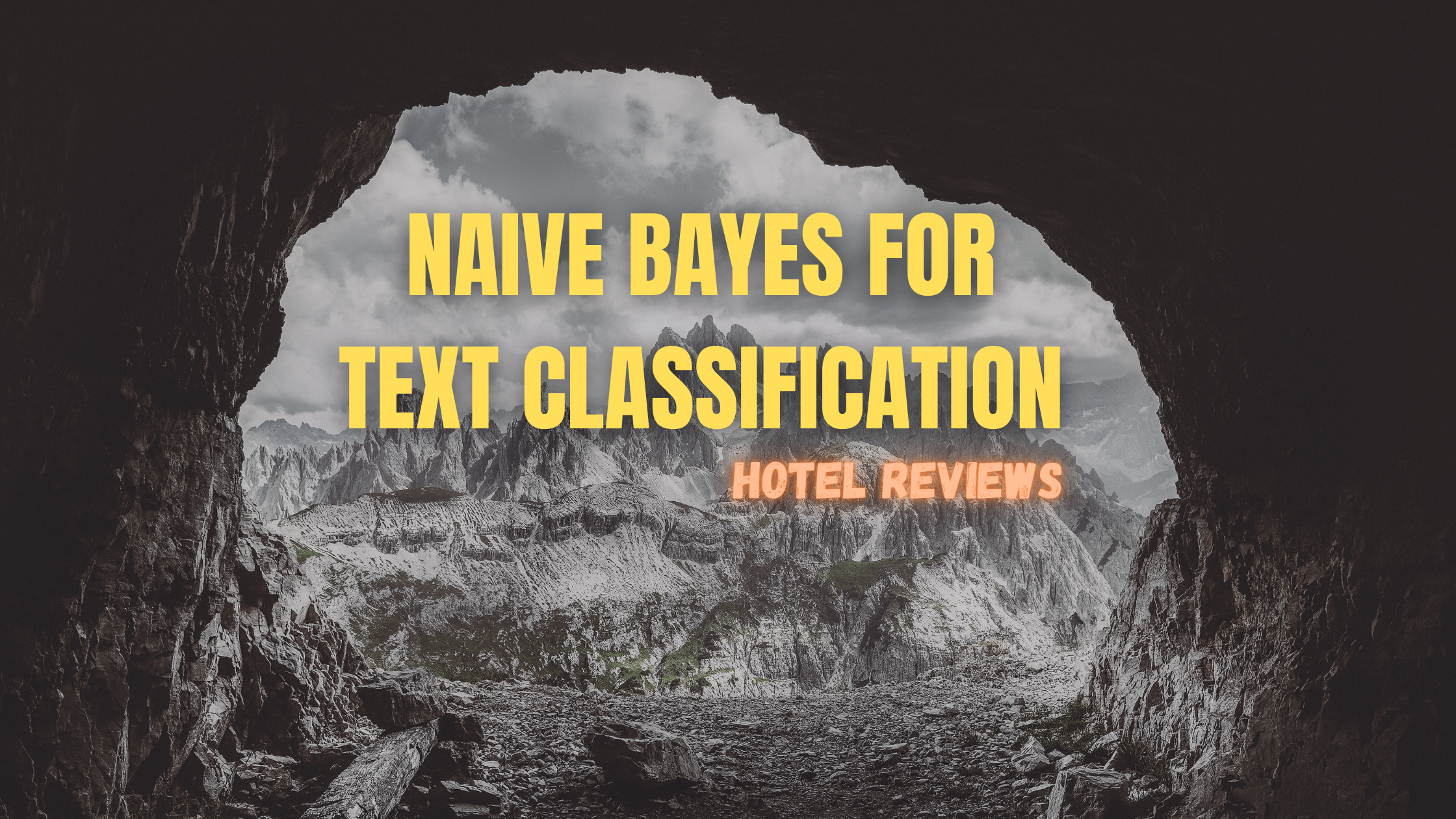# Naive Bayes For Text ClassificationFirst we calculate the probability for the review to be positive.

p(y=positive/x1,x2…xn) = p(y)*p(x1/y)*p(x2/y) where x1, x2 are the words present in review 1 after all the text preprocessing. x1 = food and x2 = good.

p(x1/y) = number of times x1(food) occurs when y is positive(1) / total number for y or len(y)

p(x1/y) = 3/5

same we can calculate for p(x2/y) = 1

then for p(y) = number of times positive(1) occurs in y / total number of y

p(y) = 3/5

so,

p(y=positive/x1,x2….xn) = 3/5*3/5*1 = 9/25 = 0.36

p(y=positive/x1,x2….xn)=0.36

Now we will calculate for

p(y=negative/x1,x2….xn) = p(y)*p(x1/y)*p(x2/y)

p(y) = number of times Negative(0) occurs in y / total number of y

p(y) = 2/5

p(x1/y) = 2/5

p(x2/y) = 0

so, p(y=negative/x1,x2….xn) = 2/5*2/5*0

p(y=negative/x1,x2….xn) = 0

Probability for p(y=positive) is 0.36 and probability of p(y=negative) is 0.

y = argmax(p(y=negative/x1,x2….xn) p(y=positive/x1,x2….xn))
y = argmax(0 0.36)
y = 1,Positive

The argmax of both the probability is 1 means positive. according to naive bayes review 1 is positive.

Let’s try for new review = {the food is tasty. I loved it.}

First we need to do some text preprocessing on to text after that we convert it into bag of words then we apply our algorithm.

Bage Of Words Representation of new review is : {10110}

Try for new review = {Food is good but taste bad}

Remember that for taste we need to add one more column.

## Conclusion

Naive Bayes works very well when used in text classification. One of the best advantage of naive bayes algorithm is also performs well when the training data is much larger or have some missing values. In naive bayes we don’t have to handle the missing values it automatically handles the missing values by just skipping the instance.

“If somewhere in NLP we stuck thinking which algorithm to apply just apply Naive Bayes because it always works well”